# Comparing fractions on a number line | Fractions | 4th grade | Khan Academy

##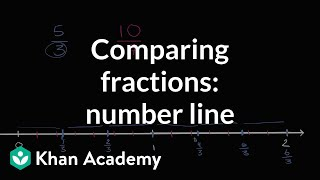By Khan Academy

Comparing fractions on a number line# Equivalent fractions on the number line

##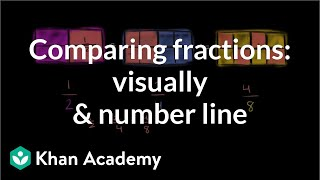By Khan Academy

Compare fractions by graphing them a number line and drawing fraction models.# Equivalent fractions on the number line

##By Khan Academy

Compare fractions by graphing them a number line and drawing fraction models.# Equivalent fractions on the number line

##By Khan Academy

Compare fractions by graphing them a number line and drawing fraction models.# Equivalent fractions on the number line

##By Khan Academy

Compare fractions by graphing them a number line and drawing fraction models.# Plot Fractions on the Number Line

##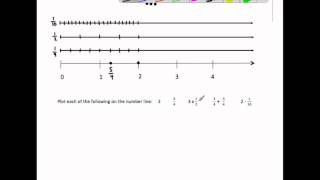By MathwithMrAlmeida

This video plots 6 fractions and operations with fractions on the number line. It ends by plotting all the same fractions on the number line and identify a pair of equivalent fractions and comparing each number's distance from zero.# Math Antics - Types of Fractions

##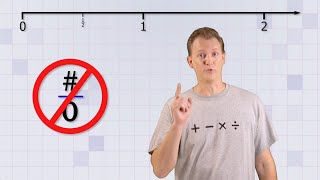By mathantics# Decimals on the number line 2

##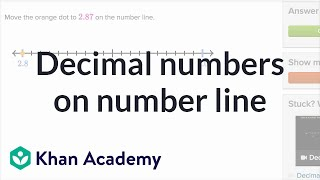By Khan Academy

Learn how to find decimals with hundredths on a number line.# Number line 1 | Multiplication and division | Arithmetic

##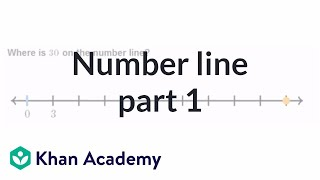By Khan Academy

Whole numbers on the number line# Equivalent Fractions How To Teach Common Core Math to 3rd or 4th Graders

##By Promoting Success# 07 - Writing & Comparing Fractions - (Equivalent Fractions & More) - Part 1

##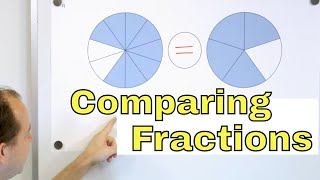By Math and Science

Quality Math And Science Videos that feature step-by-step example problems!##By Anywhere Math# Rational Numbers

##By Anywhere Math# 01 - What are Equivalent Fractions? - (Calculate & Find Equivalent Fractions) - Part 1

##By Math and Science

Quality Math And Science Videos that feature step-by-step example problems!# Integers and Absolute Values

##By Anywhere Math# Graphing Fractions and Decimals on the Number Line

##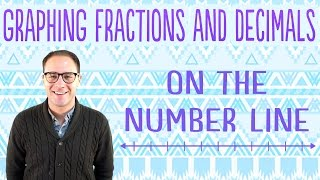By Anywhere Math# Math Antics - Converting Any Fraction

##By mathantics# Math Antics - Convert any Fraction to a Decimal

##By mathantics# Slope

##By Anywhere Math# Math Antics - Measuring Distance

##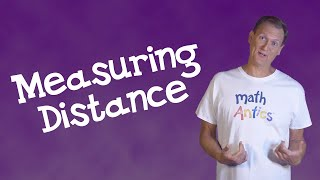By mathantics